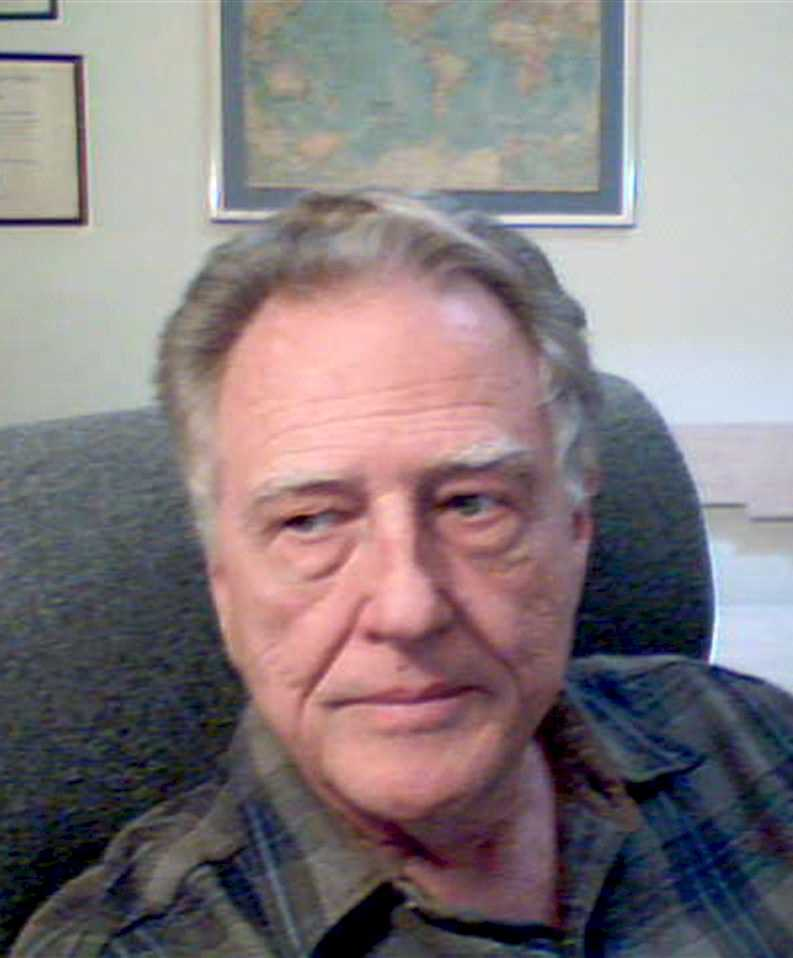Enter the content which will be displayed in sticky bar

MemberJames Keele
local time: 2019-06-17 20:58 (-06:00 DST)
James Keele Abstracts
Titles
• Force and Rotation (2010) [Updated 8 years ago]
• [Updated 2 years ago]
• [Updated 7 years ago]
• [Updated 2 years ago]
• [Updated 6 years ago]
• [Updated 6 years ago]
• [Updated 6 years ago]
• [Updated 2 years ago]

• Abstracts Details
• Force and Rotation (2010) [Updated 8 years ago]

This paper establishes a relation between what the author defines as Energy Moment and electrostatic force. The charge e is defined with fundamental constants and is shown to be closely related to the energy of the particle and a rotational radius value. The standard Coulomb Law for force between charges such as an electron and a proton is shown to exist in a form different from what is customarily presented in physics. New insights into the nature of forces are gained from the new force equations.

• [Updated 2 years ago]

This paper summarizes the derivation of SRT electrodynamics laws for relatively moving charges. These laws are based on Coulomb's Law and SRT, both of which have been basic in conventional physics for over 100 years. Relativists have determined an equation for the transform of the electric field of a relatively moving electric charge. However, that transform is usually based on a transform of the Lorentz Force Law. Part of the Lorentz Force law was found to be unnecessary to derive the magnetic force on a stationary charge due to a moving charge. Several electrodynamics laws emerge from this transform: The ?cardinal law? as described by Maxwell, the law for the force between slow relatively moving charges, the law between a stationary charge and a current element, similar to Gauss's Law, and the old law for force between current elements as determined by Amp?re in 1822. An experiment was performed to check on the validity of the old Amp?re's Law.

• [Updated 7 years ago]

The reason that a finite length current element may legitimately be used in the mathematics of calculating forces and energy between current carrying wires is because the current carrying element has canceling stored energy in it. This fact is demonstrated by an experiment conducted by the author. This experiment measured the inductance of several different shaped single turn coils. The measured value of inductance was then compared to the value of inductance calculated employing the integral of Ampere?s Law. It was discovered that by adjusting the length of the current element a match between the measured and calculated inductance could be obtained for all the single turn coils. The inductance of the current element, itself, was then calculated using the integral of Ampere?s Law. The inductance of the length required for the match was found to be zero. There are mechanisms in the current element that give up energy to supply the energy stored in it so that the net stored energy is zero.

• [Updated 2 years ago]
It is difficult to find critical work about Einstein's Theory of Relativity in most standard physics journals. Galilean Electrodynamics, founded by the late Dr. Petr Beckmann in 1989, is a notable exception. Since Einstein's 1905 paper, Relativity has had many critics and although it is widely accepted today, there is still a minority who question the central tenets of Relativity Theory. Galilean Electrodynamics is devoted to publishing high quality scientific papers, refereed by professional scientists, that are critical of Special Relativity, General Relativity, Quantum Mechanics, Big Bang theory and other establishment doctrines.

• [Updated 6 years ago]

In conventional SRT, mass is said to increase with speed according to the factor g in the equation m = g x mo. Also, the energy of mass m is expressed by E = mc2. It is customary to identify E in this latter equation as the combination of rest energy and kinetic energy. This paper examines the implication of assuming E also includes the particle's potential energy, and the additional implication of 'potential mass' in all m = E/c2. The Bohr Atom Theory is used for a test.

• [Updated 6 years ago]

Writing in a recent issue of Galilean Electrodynamics, Osborne and Pope proposed, and to some extent demonstrated, that all forces are due to conservation of angular momentum, and not due to gravity, electrostatic, inertia, or other forces. The central idea is that material objects, neutral mass, and charged mass, when moving in a 'natural' orbit about another body, experience no forces. Also presented was the idea that orbits, and not rectilinear motions, are the natural motions of particles. While Pope and Osborne developed the idea somewhat at the macro and micro levels, the author of the present paper re-examines these ideas at the micro level in a slightly different manner, using the Bohr Atom. What is demonstrated is that the electrical forces associated with Coulomb's Law can be attributed to angular momentum or kinetic energy moments, instead of electric charges.

• [Updated 6 years ago]

This paper shows that the potential energy set up by the Amp?re force between current elements is the mechanism for energy storage in inductors. The use of Amp?re's Law , without reference to a 'magnetic field', explains energy storage in the simple inductors experimented tested and reported upon in this paper. The paper shows how to develop a computer model for a one-turn inductor and calculate the energy stored in the inductor.

• [Updated 2 years ago]

Andre Ampere experimentally determined in 1822 a law that describes the force of interaction between two current elements. This paper theoretically derives this law from Special Relativity Theory (SRT). Also, the derivation shows the correct law for force of interaction between two relatively moving isolated charges. In addition, the derivation shows there is a force between a stationary charge and a current element.